﻿ Using Differentiation Term by Term Theorem to Study the Partial Differential Problems

### Using Differentiation Term by Term Theorem to Study the Partial Differential Problems

Chii-Huei YuOPEN ACCESSPEER-REVIEWED

## Using Differentiation Term by Term Theorem to Study the Partial Differential Problems

Department of Management and Information, Nan Jeon University of Science and Technology, Tainan City, Taiwan

### Abstract

This article takes the mathematical software Maple as the auxiliary tool to study the partial differential problem of two types of multivariable functions. We can obtain the infinite series forms of any order partial derivatives of these two types of functions by using differentiation term by term theorem, and hence greatly reduce the difficulty of calculating their higher order partial derivative values. On the other hand, we propose two examples to do calculation practically. The research methods adopted in this study involved finding solutions through manual calculations and verifying our answers by using Maple.

• Yu, Chii-Huei. "Using Differentiation Term by Term Theorem to Study the Partial Differential Problems." Turkish Journal of Analysis and Number Theory 1.1 (2013): 63-68.
• Yu, C. (2013). Using Differentiation Term by Term Theorem to Study the Partial Differential Problems. Turkish Journal of Analysis and Number Theory, 1(1), 63-68.
• Yu, Chii-Huei. "Using Differentiation Term by Term Theorem to Study the Partial Differential Problems." Turkish Journal of Analysis and Number Theory 1, no. 1 (2013): 63-68.

 Import into BibTeX Import into EndNote Import into RefMan Import into RefWorks

### 1. Introduction

The computer algebra system (CAS) has been widely employed in mathematical and scientific studies. The rapid computations and the visually appealing graphical interface of the program render creative research possible. Maple possesses significance among mathematical calculation systems and can be considered a leading tool in the CAS field. The superiority of Maple lies in its simple instructions and ease of use, which enable beginners to learn the operating techniques in a short period. In addition, through the numerical and symbolic computations performed by Maple, the logic of thinking can be converted into a series of instructions. The computation results of Maple can be used to modify our previous thinking directions, thereby forming direct and constructive feedback that can aid in improving understanding of problems and cultivating research interests.

In calculus and engineering mathematics curricula, the evaluation and numerical calculation of the partial derivatives of multivariable functions are important. For example, Laplace equation, wave equation, as well as other important physical equations are involved the partial derivatives. On the other hand, evaluating the m-th order partial derivative value of a multivariable function at some point, in general, needs to go through two procedures: firstly determining the m-th order partial derivative of this function, and then taking the point into the m-th order partial derivative. These two procedures will make us face with increasingly complex calculations when calculating higher order partial derivative values ( i.e. m is large), and hence to obtain the answers by manual calculations is not easy. In this paper, we study the partial differential problem of the following two types of n-variables functions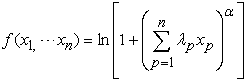(1)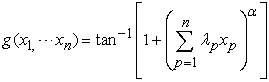(2)

where α > 0, n is a positive integer, and λp are real numbers for all p = 1, , n. We can obtain the infinite series forms of any order partial derivatives of these two types of multivariable functions using differentiation term by term theorem; these are the major results of this study (i.e., Theorem 1 and Theorem 2), and hence greatly reduce the difficulty of calculating their higher order partial derivative values. As for the study of related partial differential problems can refer to [1-13]. On the other hand, we provide two examples to do calculation practically. The research methods adopted in this study involved finding solutions through manual calculations and verifying these solutions by using Maple. This type of research method not only allows the discovery of calculation errors, but also helps modify the original directions of thinking from manual and Maple calculations. Therefore, Maple provides insights and guidance regarding problem-solving methods.

### 2. Main Results

Firstly, we introduce some notations and formulas used in this study.

2.1. Notations

2.1.1. Suppose n is a positive integer, are λp real numbers for all p = 1, , n. Defineand2.1.2. Suppose r is any real number, m is any positive integer. Define2.1.3. Suppose n is a positive integer, jp are non-negative integers for all p = 1, , n. For the n-variables function f (x1, x2, , xn), its jp-times partial derivative with respect to xp for all p = 1, , n, forms a j1 + j2 + + jn-th order partial derivative, and denoted by2.2. Formulas

2.2.1.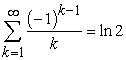.

2.2.2..

Next, we introduce an important theorem used in this paper.

2.3. Differentiation Term by Term Theorem ()

For all non-negative integers k, if the functions gk (a, b) → R satisfy the following three conditions：(i) there exists a point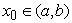such that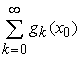is convergent, (ii) all functions gk (x) are differentiable on open interval (a, b), (iii)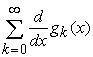is uniformly convergent on (a, b). Thenis uniformly convergent and differentiable on (a, b). Moreover, its derivative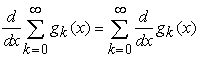.

Before deriving the first major result in this study, we need a lemma.

2.4. Lemma 1

Suppose y is a real number with y > -1. Then the logarithm function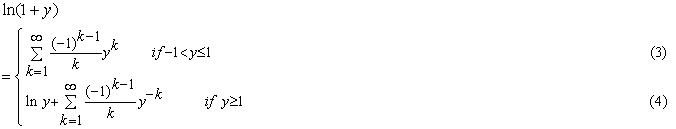Proof If -1 < y < 1, because the derivative of ln (1 + y),(5)

By Formula 2.2.1, we obtainfor all -1 < y ≤ 1.

If y ≥ 1, then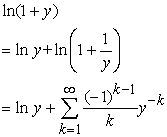for all y ≥ 1.

Next, we determine the infinite series forms of any order partial derivatives of the n-variables function (1).

2.5. Theorem 1

Suppose n is a positive integer, α > 0, and λp are real numbers, jp are non-negative integers for all p = 1, , n. If the n-variables function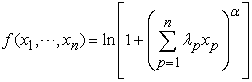satisfies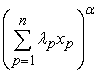exists,, and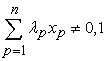.

Case(1) If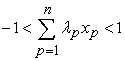, then the j1 + j2 + + jn-th order partial derivative of f (x1, , xn),(6)

Case(2) If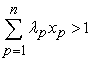, then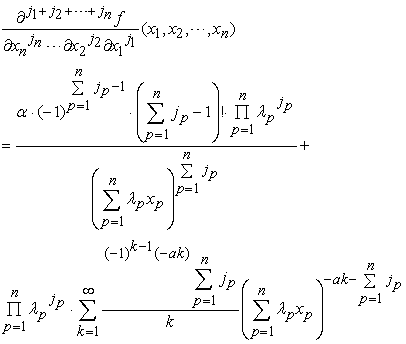(7)

Proof Case(1) If, because(8)

(By Eq. (3))

By differentiation term by term theorem, differentiating jp-times with respect to xp for all p = 1, , n on both sides of Eq. (8), we obtainCase(2) If, by Eq. (4),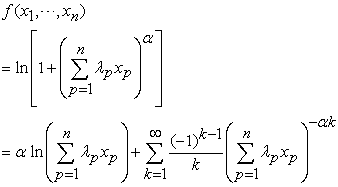(9)

Also, by differentiation term by term theorem, differentiating jp-times with respect to xp for all p = 1, , n on both sides of Eq. (9), we obtain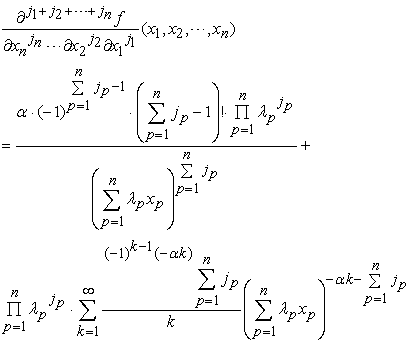Before deriving the second major result in this paper, we also need a lemma.

2.6. Lemma 2

Suppose y is a real number. Then the inverse tangent functionProof The derivative of tan-1 y,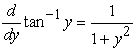If -1<y<1, then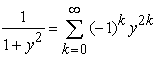(13)

By Formula 2.2.2 , we obtain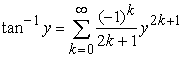for all -1 ≤ y ≤ 1.

If, because(14)

By Formula 2.2.2, we have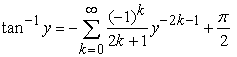for all y ≥ 1.

Andfor all y ≤ -1.

Finally, we find the infinite series forms of any order partial derivatives of the n-variables function (2).

2.7. Theorem 2

If the assumptions are the same as Theorem 1. Assume the n-variables function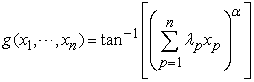satisfiesexists, and.

Case(1) If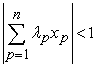, then the j1 + j2 + + jn-th order partial derivative of g (x1, , xn),(15)

Case(2) If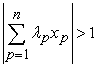, then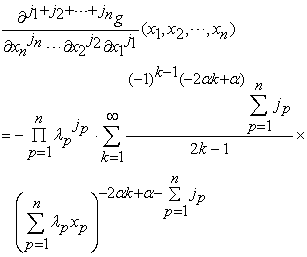(16)

Proof

Case(1) If, by Eq. (10), we have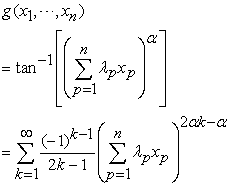(17)

Using differentiation term by term theorem, differentiating jp-times with respect to xp for all p = 1, , n on both sides of Eq. (17), we haveCase(2) If, by Eqs. (11) and (12), and using differentiation term by term theorem, we obtain### 3. Examples

In the following, for the partial differential problem of the two types of multivariable functions in this study, we provide two examples and use Theorems 1, 2 to determine the infinite series forms of any order partial derivatives and some higher order partial derivative values of these functions. In addition, we employ Maple to calculate the approximations of these higher order partial derivative values and their solutions for verifying our answers.

3.1. Example 1

Suppose the two-variables function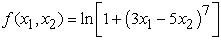(18)

satisfies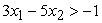and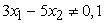.

Case (1) If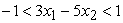, then by Eq. (6), we obtain any j1 + j2-th order partial derivative of f (x1, x2),(19)

Thus, we can determine the 12-th order partial derivative value of f (x1, x2) at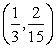,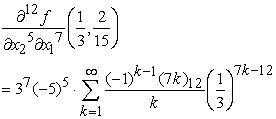(20)

Next, we use Maple to verify the correctness of Eq. (20).

>f:=(x1,x2)->ln(1+(3*x1-5*x2)^7);

>evalf(D[1\$7,2\$5](f)(1/3,2/15),18);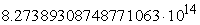>evalf(3^7*(-5)^5*sum((-1)^(k-1)*product(7*k-j,j=0..

11)/k*(1/3)^(7*k-12),k=1..infinity),18);Case (2) If, using Eq. (7), we obtain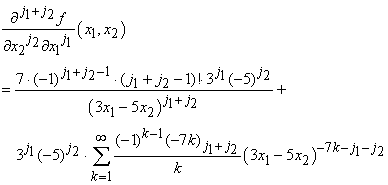(21)

Hence, we obtain the 14-th order partial derivative value of f (x1, x2)at,(22)

>evalf(D[1\$6,2\$8](f)(2,4/5),18);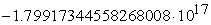>evalf(-7*13!*3^6*(-5)^8/2^14+3^6*(-5)^8*sum((-1)^(k-1)*product(-7*k-j,j=0..13)/k*2^(-7*k-14),k=1..infinity),

18);3.2. Example 2

Assume the three-variables function(23)

satisfies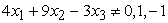.

Case (1) If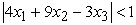, using Eq. (15), we obtain any j1 + j2 + j3-th order partial derivative of g (x1, x2, x3),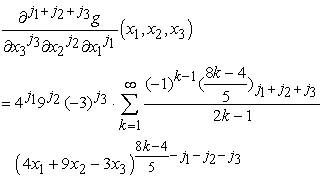(24)

Thus, we can evaluate the 15-th order partial derivative value of g (x1, x2, x3) at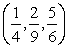,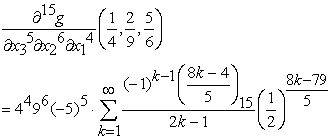(25)

We also use Maple to verify the correctness of Eq. (25).

>g:=(x1,x2,x3)->arctan((4*x1+9*x2-3*x3)^(4/5));

>evalf(D[1\$4,2\$6,3\$5](g)(1/4,2/9,5/6),18);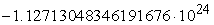>evalf(4^4*9^6*(-3)^5*sum((-1)^(k-1)*product((8*k-4)/5-j,j=0..14)/(2*k-1)*(1/2)^((8*k-79)/5),k=1..

infinity),18);Case (2) If, by Eq. (16), we have(26)

Thus, we obtain the 17-th order partial derivative value of g (x1, x2, x3) at (3, 1, 6),(27)

>evalf(D[1\$7,2\$4,3\$6](g)(3,1,6),18);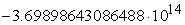>evalf(-4^7*9^4*(-3)^6*sum((-1)^(k-1)*product((-8*k+4

)/5-j,j=0..16)/(2*k-1)*3^((-8*k-81)/5),k=1..infinity),18);### 4. Conclusion

In this paper, we provide a new technique to evaluate any order partial derivatives of two types of multivariable functions. We hope this technique can be applied to solve another partial differential problems. On the other hand, the differentiation term by term theorem plays a significant role in the theoretical inferences of this study. In fact, the applications of this theorem are extensive, and can be used to easily solve many difficult problems; we endeavor to conduct further studies on related applications. In addition, Maple also plays a vital assistive role in problem-solving. In the future, we will extend the research topic to other calculus and engineering mathematics problems and solve these problems by using Maple. These results will be used as teaching materials for Maple on education and research to enhance the connotations of calculus and engineering mathematics.

### References

  L. E. Fraenkel, Formulae for high derivatives of composite functions, Mathematical Proceedings of the Cambridge Philosophical Society, Vol. 83, pp. 159-165, 1978.In article CrossRef  T-W, Ma, Higher chain formula proved by combinatorics, The Electronic Journal of Combinatorics , Vol. 16, #N21, 2009.In article  D. N. Richard, An efficient method for the numerical evaluation of partial derivatives of arbitrary order, ACM Transactions on Mathematical Software, Vol. 18, No. 2, pp. 159-173, 1992.In article CrossRef  C. H., Bischof, G. Corliss, and A. Griewank, Structured second and higher-order derivatives through univariate Taylor series, Optimization Methods and Software, Vol. 2, pp. 211-232, 1993.In article CrossRef  A. Griewank and A. Walther, Evaluating derivatives: principles and techniques of algorithmic differentiation, 2nd ed., SIAM, Philadelphia, 2008.In article CrossRef  C. -H. Yu, Using Maple to evaluate the partial derivatives of two-variables functions, International Journal of Computer Science and Mobile Computing, Vol. 2, Issue. 6, pp. 225-232, 2013.In article  C.-H. Yu, Using Maple to study the partial differential problems, Applied Mechanics and Materials, in press, 2013.In article  C. -H. Yu, Evaluating partial derivatives of two-variables functions by using Maple, Proceedings of the 6th IEEE/International Conference on Advanced Infocomm Technology, Taiwan, pp.23-27, 2013.In article  C.-H. Yu, Application of Maple: taking the partial differential problem of some types of two-variables functions as an example, Proceedings of the International Conference on e-Learning, Taiwan, pp. 337-345, 2013.In article  C.-H. Yu, Application of Maple on the partial differential problem of four types of two-variables functions, Proceedings of the International Conference on Advanced Information Technologies, Taiwan, No.87, 2013.In article  C.-H., Yu, Application of Maple: taking the partial differential problem of two-variables functions as an example, Proceedings of 2013 Business Innovation and Development Symposium, Taiwan, B20130113001, 2013.In article  C. -H. Yu and B. -H. Chen, The partial differential problem, Computational Research, Vol.1, No.3, pp.53-60, 2013.In article  C. -H. Yu, Partial derivatives of some types of two-variables functions, Pure and Applied Mathematics Journal , Vol. 2, No. 2, pp. 56-61, 2013.In article CrossRef  T. M. Apostol, Mathematical analysis, 2nd ed., Addison-Wesley, Boston, p230, 1975.In article